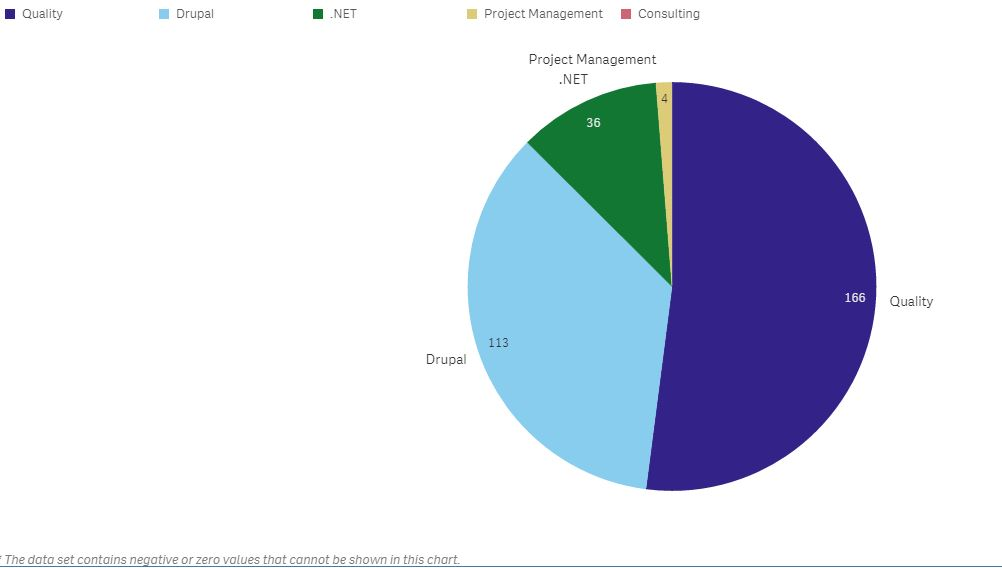New to Qlik Sense

Discussion board where members can get started with Qlik Sense.

Highlighted
Contributor II

How to Create Pie Chart With two measures in Qlik Sense

Hi Guys,

I have a requirement, I want to create pie char/ donut chart with two measures below i am mentioning my dimensions & measures

Dimension: Skill Name

Expression1: =Sum({<[Closed date] = {"\$(='>=' & Date(Today()-30))"},[Job Status]={Closed}>} [Total No. of Openings])

Expression2: ==Sum({<[Closed date] = {"\$(='>=' & Date(Today()-30))"},[Job Status]={Closed}>} [Ageing in days])

I want my chart like this.. this chart i created in qlikview

I legend i have mentioned dimension and Expression1 and in chart Expression2 values are visiblingRegards,

B V S Sudhakar

3 RepliesMVP

Re: How to Create Pie Chart With two measures in Qlik Sense

Yes you can do it in Qlik Sense too - but it has to be done in an entirely different way as you can't have more than one measure. However this single measure can use the Dual() to concatenate several expressions into a string part while providing only the original measure into the numeric part of the Dual().In addition you will have to set the measure's "Number formatting" to "Measure expression" and then under "Appearance", "Presentation" switch the "Value Labels" to "Custom" and select "Values".

Contributor II

Re: How to Create Pie Chart With two measures in Qlik Sense

Hi,

I tried with Dual Expression:

dual(Sum({<[Closed date] = {"\$(='>=' & Date(Today()-30))"},[Job Status]={Closed}>} [Total No. of Openings]),Sum({<[Closed date] = {"\$(='>=' & Date(Today()-30))"},[Job Status]={Closed}>} [Ageing in days]))

I got the chart like this:But i want one change, I want no.of openings values in legend (Eg: Quality  1, Drupal   4, .NET 2.... like this).

In chart i have show Ageing days but in legend i want openings values.

And

Is there any Possibility to show null/zero values in this chart

Contributor II